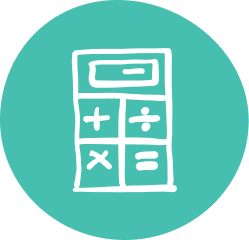### Quality Grade 8 Math Tutoring & Help

Oxford Learning®’s 8th Grade math tutoring program is specifically designed to help students improve their learning by giving them a thorough understanding of all the components of the course and igniting a passion for learning.

Your teen’s Grade 8 math tutor not only teaches math concepts, but also teaches active thinking and math confidence, both essential skills for math success.

At Oxford Learning®, learning is about more than better grades. Our cognitive approach helps teens learn how to learn, a skill that transfers from classroom to classroom. Our personalized Grade 8 math tutoring gives teens the confidence to take their math skills to the next level, for math success this year and every year.

### Grade 8 Math Tutoring Subjects

##### Number Sense/Numeration
• Representing and ordering rational numbers
• Representing numbers using exponential notation
• Solving multi-step problems involving whole numbers and decimals
• Multiplying and dividing fractions and integers
• Multiplying and dividing decimals by the power of 10
• Applying orders of operations using brackets and exponents
• Solving problems involving percentages to one decimal place and percentages greater than 100
• Solving problems involving rates and proportions
##### Measurement
• Convert from cubic centimetres and metres to millilitres and cubic centimetres
• Develop circumference and area relationships in a circle
• The formula for the volume of a cylinder
• Surface area relationships for cylinders
##### Geometry & Spatial Sense
• Sorting quadrilaterals by geometric properties involving diagonals
• Constructing circles
• Investigating relationships among similar shapes
• Determining and applying angle relationships for parallel and intersecting lines
• Relating the number of faces
• Edges and vertices of a polyhedron
• The Pythagorean relationship geometrically
• Plotting the image of a point on the coordinate plane after applying a transformation
##### Algebra & Patterning
• Representing the general term in a linear sequence
• Using one or more algebraic expressions
• Translating statements using algebraic equations
• Finding the term number in a pattern algebraically when given any term
• Solving linear equations involving one-variable terms with integer solutions using a ‘balance’ model
##### Data Management & Probability
• Collecting categorical, discrete and continuous data
• Organizing data into intervals
• Displaying data using histograms and scatter plots
• Using measures of central tendency to compare sets of data
• Comparing two attributes using data management tools
• Comparing experimental and theoretical probabilities
• Calculating the probability of complementary elements